🛰️

# Baseline Model in Super-resolution of City Images

2021/11/07に公開

## Overview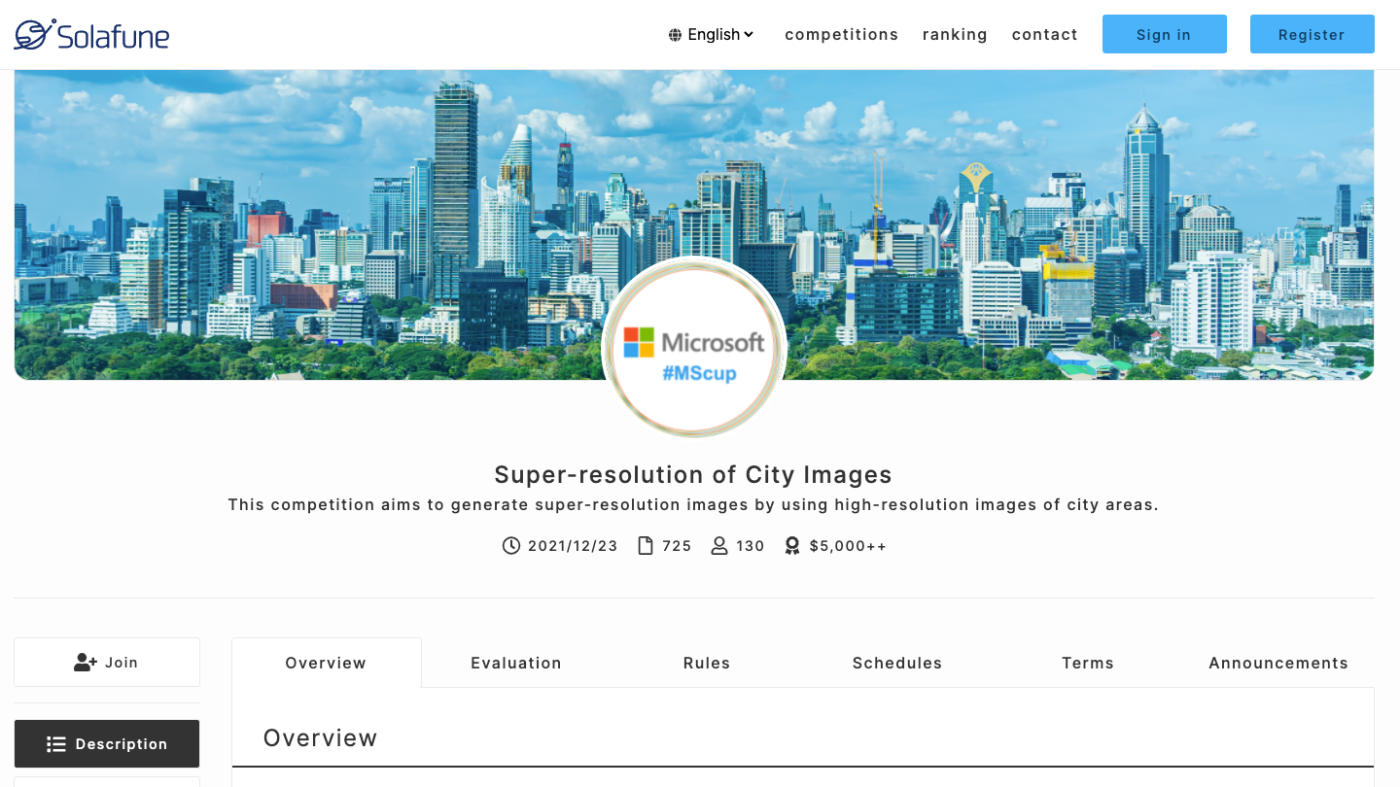An example of super-resolution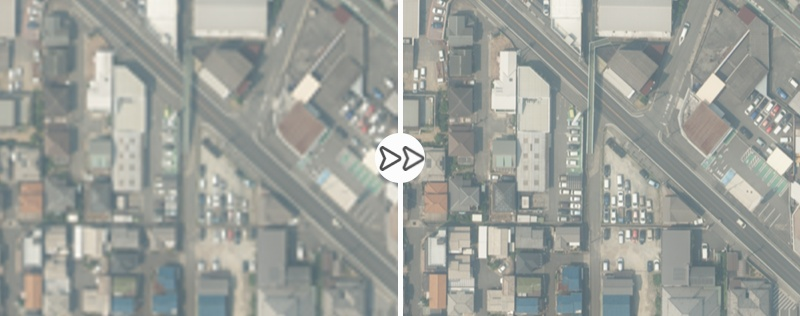⚠️
`cf. @solafune(https://solafune.com) `

``````Use for any purpose other than participation in the competition or commercial use is prohibited.
``````

## Algorithms

The algorithm we use is ESPCN. To introduce this algorithm, we will first explain the use of deep learning in a single image super-resolution.

### SRCNN

As the name suggests, this algorithm applies CNN to the super-resolution task. It resizes a low-resolution image to the same size as a high-resolution image and passes it through 3 to 5 layers of CNN to increase the resolution.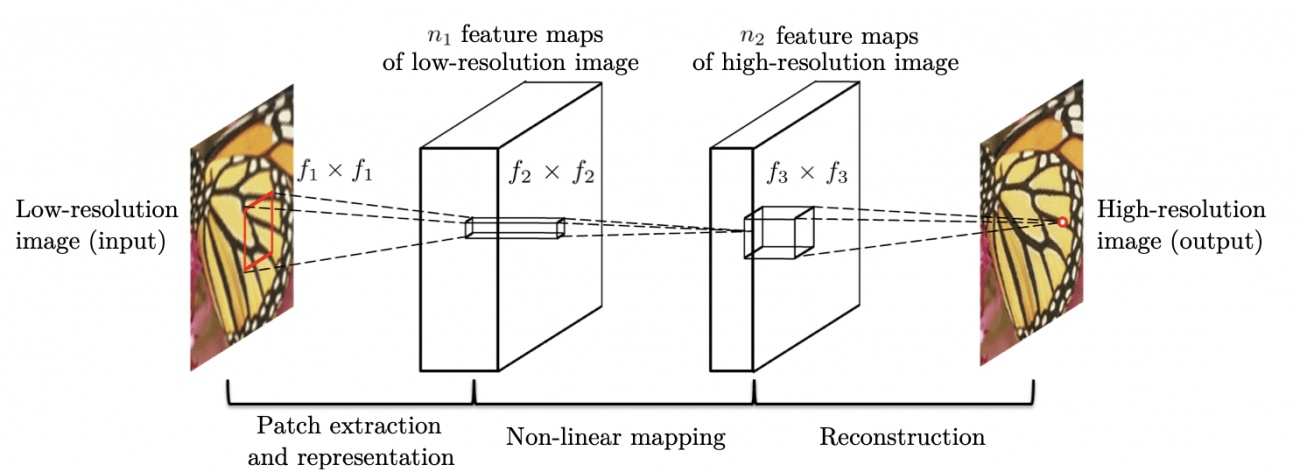source:https://arxiv.org/pdf/1501.00092.pdf

### FSRCNN

This algorithm was invented by the developers of SRCNN to spped it up. In SRCNN, the need to resize low-resolution images beforehand has been an issue for time improvement. Therefore, FSRCNN increases the resolution by performing deconvolution on the last layer of the neural network to solve this issue.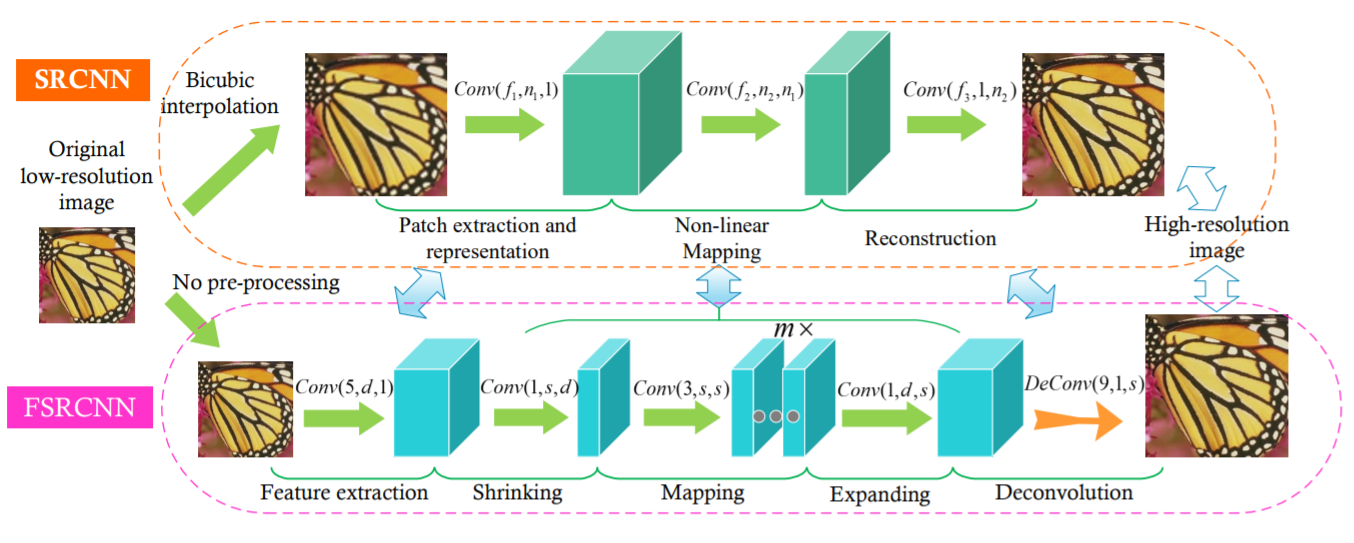source:https://arxiv.org/pdf/1608.00367.pdf

### ESPCN

FSRCNN used deconvolution to increase the resolution, but the generated image may show speckled patterns. To improve the images, ESPCN uses an algorithm called Sub-Pixel Convolution (Pixel Shuffle) instead of deconvolution.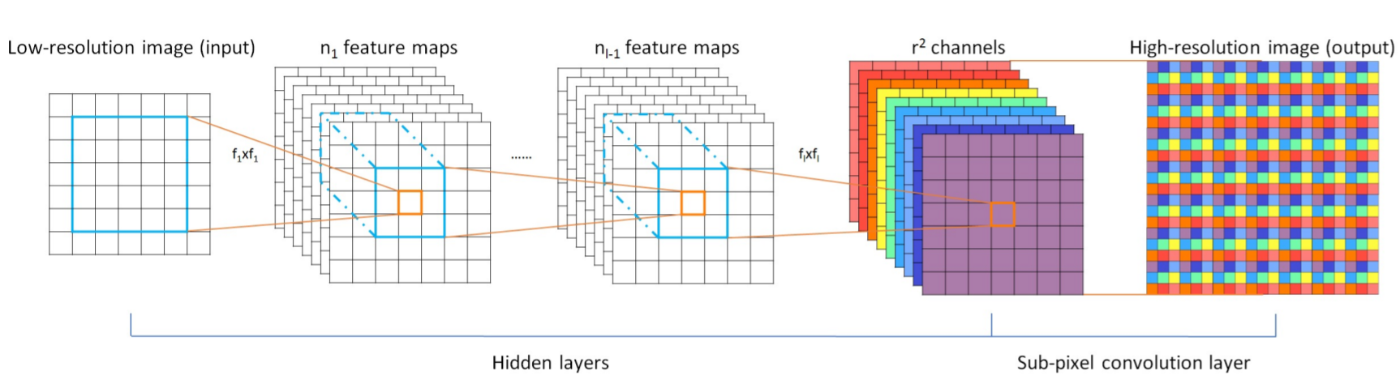source:https://arxiv.org/pdf/1609.05158.pdf

This algorithm has had a significant impact on the development of later algorithms, such as SRResnet.

# Baseline

The program we used follows Apache License 2.0.
The ESPCN referred to is also available under the Apache License 2.0.

``````Copyright 2021 @Solafune

you may not use this file except in compliance with the License.
You may obtain a copy of the License at

Unless required by applicable law or agreed to in writing, software
WITHOUT WARRANTIES OR CONDITIONS OF ANY KIND, either express or implied.
See the License for the specific language governing permissions and
``````

## Directory structure

``````┣━ train
┃　　┗━ train_*.tif
┣━ evaluation
┃　　┗━ evaluation_*.tif
┣━ output
┃　　┗━ test_*.tif
┗━ ESPCN_sample.py
``````

## Development Configuration

• Computer specs
• CPU: Intel Core i5-6500
• RAM: 8GB
• GPU: Geforce GTX 1070
• Development environment
• We used Docker to develop the environment. Dockerfile is as follows:
``````FROM tensorflow/tensorflow:latest-gpu
RUN pip install Pillow
``````
• We also checked that this also works on Google Colaboratory. In this case, you need to add/change the code for the file path and Google Drive mount.

## Code Description

### Libraries

You import the required libraries.

``````import os

os.environ["TF_FORCE_GPU_ALLOW_GROWTH"]= "true"

import tensorflow as tf
import tensorflow.keras.layers as kl
from tensorflow.python.keras import backend as K
import numpy as np
from PIL import Image
``````

### Model

This is the ESPCN model to be used in the baseline. ESPCN performs upsampling using Sub-Pixel Convolution, which is not included in Tensorflow by default, so you need to implement it by yourself.

``````# ESPCN
class ESPCN(tf.keras.Model):
def __init__(self, input_shapes):
super().__init__()

self.input_shape_lm = ( None, input_shapes, input_shapes, 3)
self.upsampling_scale = 4

self.conv_0 = kl.Conv2D(64, 5, padding="same", activation="relu", input_shape=self.input_shape_lm)
self.conv_1 = kl.Conv2D(32, 3, padding="same", activation="relu")
self.pixel_shuffle = Pixel_shuffler(self.upsampling_scale, input_shapes)

def call(self, x):
conv2d_0 = self.conv_0(x)
conv2d_1 = self.conv_1(conv2d_0)
model = self.pixel_shuffle(conv2d_1)
return model

# Pixel Shuffle
class Pixel_shuffler(tf.keras.Model):
def __init__(self, upscale, input_shape):
super().__init__()

self.upscale = upscale
self.conv = kl.Conv2D(self.upscale**2 * 3, kernel_size=3, padding="same")
self.act = kl.Activation(tf.nn.relu)

# forward proc
def call(self, x):

d1 = self.conv(x)
d2 = self.act(tf.nn.depth_to_space(d1, self.upscale))

return d2
``````

### Training

We train the image using Adam for the Optimizer and MSE for the loss function.

``````# Training
class trainer(object):
def __init__(self, lr_shape, trained_model=""):
self.model = ESPCN  ( lr_shape)
loss=tf.keras.losses.MeanSquaredError(),
metrics=[self.ssim])

if trained_model != "":

def train(self, lr_imgs, hr_imgs, out_path, batch_size, epochs):

cp_callback = tf.keras.callbacks.ModelCheckpoint(out_path,
save_weights_only=True,
verbose=10)
# Training
his = self.model.fit(lr_imgs, hr_imgs, batch_size=batch_size, epochs=epochs, callbacks=[cp_callback])

print("___Training finished\n\n")

# Saving parameter
print("___Saving parameter...")
self.model.save_weights(out_path)
print("___Completed successfully\n\n")

return his, self.model

# SSIM
def ssim(self, h3, hr_imgs):
return tf.image.ssim( h3, hr_imgs, max_val=1.0)
``````

The data is normalized for the learning process. To increase the number of data, we also generate images that are flipped and mirrored and use them as teacher data.

``````# Dataset creation
def create_dataset():

print("\n___Creating a dataset...")
prc = ['/', '-', '\\', '|']
cnt = 0
training_data =[]

for i in range(60):
d = "./train/"

# High-resolution image
img = Image.open(d+"train_{}_high.tif".format(i))
flip_img = np.array(ImageOps.flip(img))
mirror_img = np.array(ImageOps.mirror(img))
img = np.array(img)
img = (tf.convert_to_tensor(img, np.float32)) / 255.0
flip_img = (tf.convert_to_tensor(flip_img, np.float32)) / 255.0
mirror_img = (tf.convert_to_tensor( mirror_img, np.float32)) / 255.0

# Low-resolution image
low_img = Image.open(d+"train_{}_low.tif".format(i))
low_flip_img = np.array(ImageOps.flip(low_img))
low_mirror_img = np.array(ImageOps.mirror(low_img))
low_img = np.array( low_img)
low_img = (tf.convert_to_tensor( low_img, np.float32)) / 255.0
low_flip_img = (tf.convert_to_tensor(   low_flip_img, np.float32)) / 255.0
low_mirror_img = (tf.convert_to_tensor( low_mirror_img, np.float32)) / 255.0

training_data.append([img,low_img])
training_data.append([flip_img,low_flip_img])
training_data.append([mirror_img,low_mirror_img])

cnt += 1

print("\n___Completed successfully\n")

random.shuffle(training_data)
lr_imgs = []
hr_imgs = []

for hr, lr in training_data:
lr_imgs.append(lr)
hr_imgs.append(hr)

return np.array(lr_imgs), np.array(hr_imgs)
``````

### Implementation

We will implement the training using the functions and classes defined above. This time, batch_size is set to 15, and epochs are set to 1400. You may need to change these depending on your environment.

``````# Loading dataset
lr_imgs, hr_imgs = create_dataset()

print("___Start training...")
Trainer = trainer(lr_imgs.shape)
his, model = Trainer.train(lr_imgs, hr_imgs, out_path="espcn_model_weight" , batch_size=15, epochs=1400)
``````

### Inference

The images used for inference are normalized in the same way as when loading the data. It means that the images resulting from the inference are also normalized, so we adjust them to be in the same format as the original images.

``````for i in range(40):
d = "./evaluation/"

# Low-resolution image
img = np.array(Image.open(d+"test_{}_low.tif".format(i)))
img = (tf.convert_to_tensor( img, np.float32)) / 255.0
img = img[np.newaxis, :, :, :]

re = model.predict(img)
re = np.reshape(re, (1200, 1500, 3))
re = re * 255.0
re = np.clip(re, 0.0, 255.0)
sr_img = Image.fromarray(np.uint8(re))

``````

## Summary

In the baseline, we used ESPCN to test the super-resolution technique. It took several hours to a day to learn, depending on the environment.
Due to the large size of the high-resolution images used in the competition, many algorithms have not been tested which require large memory to build the neural network.
Also, the score may be improved by changing the loss function, optimization method, learning coefficient, etc.
We hope this baseline helps participants more from various perspectives. Thanks!